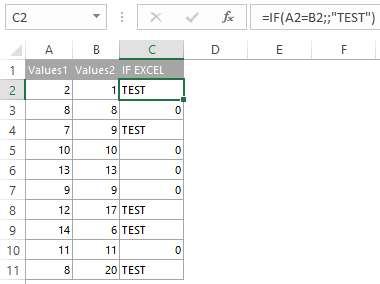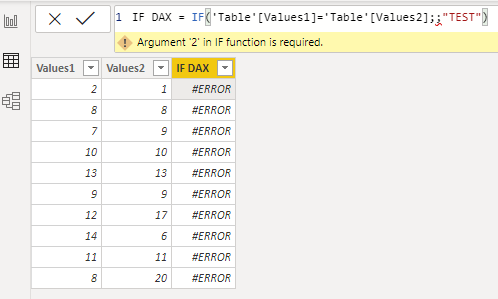# Difference between DAX and Excel functions that looks the same

If you know Excel functions, it is easier for you in Power BI with DAX. Sometimes it is mentioned as an advantage. It is indeed, but sometimes there is a significant difference between DAX and Excel functions that looks the same. I’m not talking about cell references – that are obvious.

Here are my top 3, and ill be happy if you add your examples in the comments below.

## SEARCH, FIND functions in Excel and DAX

Excel:

SEARCH(find_text,within_text,[start_num])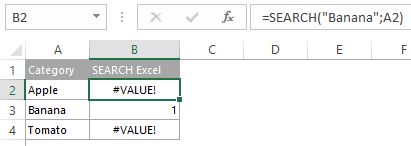DAX:

SEARCH(<find_text>, <within_text>[, [<start_num>][, <NotFoundValue>]])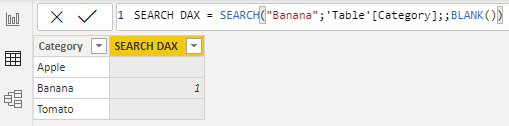In DAX the last optional argument NotFoundValue makes all the difference and that is very handy. In Excel, if SEARCH or FIND cant return any results, you get an error, and in some cases will use the IFERROR function. On the other hand, in DAX, you can use the last argument to return an appropriate value.

Great, right? Not really. Because if you do not use this optional NotFoundValue argument in DAX and some of the results are error, then all the column results are error.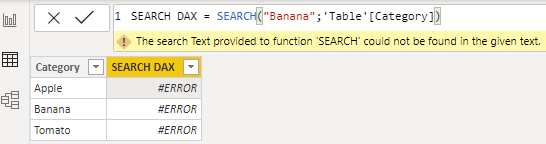## AND, OR functions in Excel and DAX

Excel

AND([logical1],[logical2],…)

DAX

AND(<logical1>,<logical2>)

As you see in Excel you can put two and more arguments in the AND function but in DAX only two. Same thing with OR function.

If I have more than 2 arguments then in Excel it goes like this.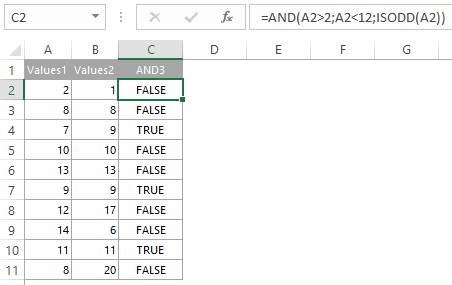If I have more than 2 arguments then in DAX  it goes like this.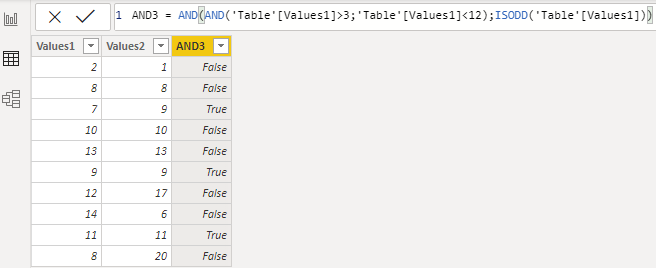Instead of AND and OR functions in DAX I recommend using && operators that means AND and || operator that means OR. By using them you are more flexible.

The same example with DAX AND logic by using && symbols goes like this.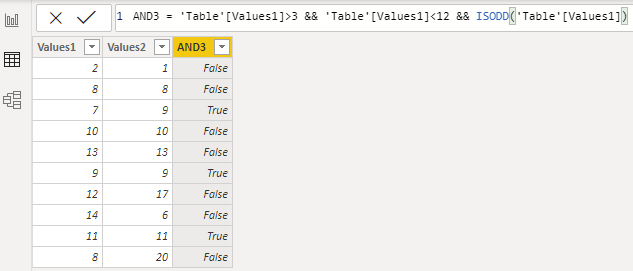## IF function in Excel and DAX

Excel

IF(logical_test, [value_if_true], [value_if_false]))

DAX

IF(<logical_test>,<value_if_true>[, <value_if_false>])

In DAX IF function has to contain value_if_true argument. It works not so flexible as in Excel, where two arguments might be optional. Here is the same example with DAX and Excel IF function.